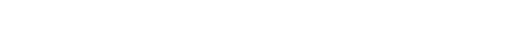## 消失的梯度问题

，相关案例代码在pylab都可以找到（我稍做了改动以支持Python3）。

``````import mnist_loader
import network2
# 输入层 784
# 隐藏层 30
# 输出层 10
sizes = [784, 30, 10]
net = network2.Network(sizes=sizes)
# 随机梯度下降开始训练
net.SGD(
training_data,
30,
10,
0.1,
lmbda=5.0,
evaluation_data=validation_data,
monitor_evaluation_accuracy=True,
)
"""
Epoch 0 training complete
Accuracy on evaluation data: 9280 / 10000
Epoch 1 training complete
Accuracy on evaluation data: 9391 / 10000
......
Epoch 28 training complete
Accuracy on evaluation data: 9626 / 10000
Epoch 29 training complete
Accuracy on evaluation data: 9647 / 1000
"""
``````

``````# 准确率 96.8%
net = network2.Network([784, 30, 30, 10])
# 准确率 96.42%
net = network2.Network([784, 30, 30, 30, 10])
# 准确率 96.28%
net = network2.Network([784, 30, 30, 30, 30, 10])
``````## 导致梯度消失的原因``````import matplotlib.pyplot as plt
import numpy as np
def sigmoid_d(x):
y = 1 / (1 + np.exp(-x))
return y * (1 - y)
x = np.arange(-4, 4, 0.1)
y = sigmoid_d(x)
plt.plot(x, y)
plt.show()
``````

Sigmoid作为激活函数情况下，由于梯度反向传播中的连乘效应，导致了梯度消失

## 在更加复杂⽹络中的不稳定梯度## 其它深度学习的障碍

```Glorot && Bengio
sigmoid
sigmoid```

```Sutskever, Martens, Dahl 和 Hinton
momentum
SGD```

Understanding the difficulty of training deep feedforward neural networks

On the importance of initialization and momentum in deep learning